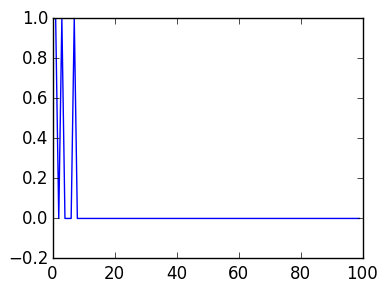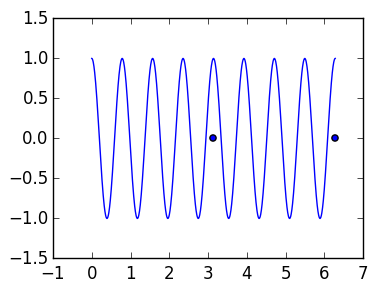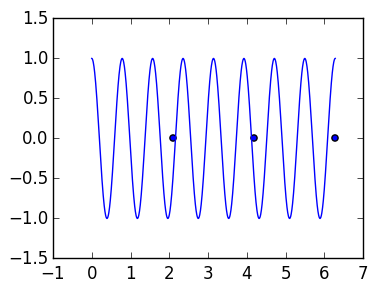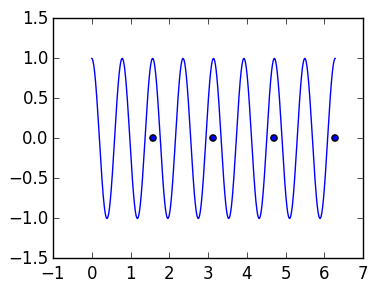# Lecture 30: The Trapezium Rule and Fourier Coefficients¶

In this lecture we start to introduce the discrete Fourier transform (DFT). The Fourier series is an expansion

$$f(\theta) = \sum_{k=-\infty}^\infty \hat f_k e^{i k \theta}$$

where

$$\hat f_k \triangleq {1 \over 2 \pi} \int_0^{2\pi} f(\theta) e^{-i k \theta} d \theta$$

We will explore calculating these coefficients using the Trapezium rule. Note that the Trapezium rule for periodic functions satisfies (since $f(2 \pi) = f(0)$)

$${2 \pi \over n} \left[{f(0) \over 2} + \sum_{j=1}^{n-1} f(\theta_j) + {f(2 \pi) \over 2} \right] = {2 \pi \over n} \sum_{j=1}^n f(\theta_j)$$

where $\theta_j = h j = {2 \pi j \over n}$. Thus define

$$Q_n[f] \triangleq {2 \pi \over n} \sum_{j=1}^n f(\theta_j)$$$$\hat f_k^n \triangleq {1 \over 2 \pi} Q_n[f(\theta) e^{-i k \theta}] = {1 \over n}\sum_{j=1}^n f(\theta_k) e^{-i k \theta_j}$$

## Observations about the first coefficient¶

The key to the DFT is based on the following observation. Consider $f(\theta) = cos 8 \theta$ and the first coefficient:

$$\hat f_0^n = {1 \over 2 \pi} Q_n[f]$$

we want this to approximate

$$\hat f_0= {1 \over 2 \pi} \int_0^{2 \pi}\cos 8 \theta d \theta = {\sin 8*2 \pi - \sin 0 \over 8} = 0.$$

In :
function trap(f,a,b,n)
h=(b-a)/n
x=linspace(a,b,n+1)

v=f(x)
h/2*v+sum(v[2:end-1]*h)+h/2*v[end]
end

Out:
trap (generic function with 1 method)

Depending on the choice of $n$, we either get the exact result, or $1$.

In :
using PyPlot
vals=Float64[trap(θ->cos(8θ),0.,2π,k)/(2π) for k=1:100]

plot(vals);Before proving this, we do an experiment to get intuition. Suppose we take two points. Then we are sampling the function at precisely the peaks of $\cos 8 \theta$:

In :
g=linspace(0.,2π,1000)

# plot the function
plot(g,cos(8g))

# plot the grid
n=2
h=2π/n
scatter(linspace(h,2π,n),zeros(n));Thus $\cos 8 \theta$ looks exactly like $1$ at the sample points, and its returning $1$. For $n=3$, on the other hand, we cancel and get zero:

In :
# plot the function
plot(g,cos(8g))

# plot the grid
n=3
h=2π/n
scatter(linspace(h,2π,n),zeros(n));But $n=4$ hits the peaks just perfectly, and again we get 1:

In :
# plot the function
plot(g,cos(8g))

# plot the grid
n=4
h=2π/n
scatter(linspace(h,2π,n),zeros(n));Proving that we get 1 for this example when we hit the frequencies just right is straightforward and intuitive. Explaining why otherwise we get exactly zero requires some effort.

# A simple sum of exponentials¶

Consider

$${1 \over 2 \pi} Q_n[e^{i k \theta}] = \sum_{j=1}^n e^{i k \theta_j}$$

for $\theta_j = {2 \pi \over n} j$. We'll show the following result that will explain the phenomenon observed above:

Theorem

$$\sum_{j=1}^n e^{i k \theta_j} = \begin{cases} n & k = \ldots,-2n,-n,0,n,2n,\ldots \cr 0 & \hbox{otherwise} \end{cases}$$

Proof

Consider $\omega \triangleq e^{i \theta_1} = e^{2 \pi i \over n}$. This is an $n$th root of unity: $\omega^n = 1$. Note that $e^{i \theta_j} =e^{2 \pi i j \over n}= \omega^j$.

(Case 1) Suppose $k$ is a multiple of $n$, that is, $k = M n$ for an integer $M$. Then we have

$$\sum_{j=1}^n e^{i k \theta_j} = \sum_{j=1}^n \omega^{kj} = \sum_{j=1}^n ({\omega^{Mn}})^j = \sum_{j=1}^n 1 = n$$

(Case 2) Recall that

$$\sum_{j=0}^{n-1} z^j = {z^n-1 \over z-1}.$$

Then we have

$$\sum_{j=1}^n e^{i k \theta_j} = \sum_{j=1}^n (\omega^k)^j = {\omega^{kn} -1 \over \omega^k -1} = 0.$$

Corollary

$${1 \over 2 \pi} Q_n[e^{i k \theta}] = \begin{cases} 1 & k = \ldots,-2n,-n,0,n,2n,\ldots \cr 0 & \hbox{otherwise} \end{cases}$$

We can thus explain the previous observation: for $f(\theta) = \cos 8 \theta$, we have

\begin{align*} \hat f_0^n &= {1 \over 2 \pi} Q_n[cos 8 \theta] = {1 \over 2 \pi} Q_n[{e^{i8 \theta} + e^{-i 8 \theta} \over 2} ] = {1 \over 4 \pi} Q_n[e^{i8 \theta}] + {1 \over 4 \pi} Q_n[e^{-i8 \theta}] \cr = \begin{cases} 1 & \hbox{ 8 is a multiple of n}\cr 0 & \hbox{otherwise} \end{cases} \end{align*}

Next lecture we will use this approach to deduce a very simple formula for $\hat f_k^n$ in terms of the true Fourier coefficients.